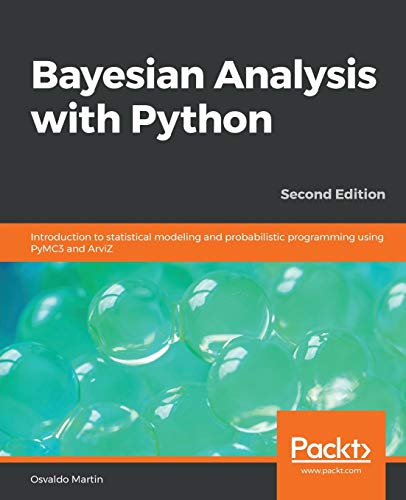Buy it at best price from here

### Rs. 747

Get Instant Cashback when you pay using Amazon Pay... Click Here

### Book Specification

 Binding Paperback Language English Number Of Pages 356 Author Osvaldo Martin Publisher Packt Publishing Limited Isbn-10 1789341655 Isbn-13 9781789341652 Dimension 19.05*2.06*23.5

# Bayesian Analysis with Python: Introduction to statistical modeling and probabilistic programming using PyMC3 and ArviZ, 2nd Edition

## Osvaldo Martin's Bayesian Analysis with Python: Introduction to statistical modeling and probabilistic programming using PyMC3 and ArviZ, 2nd Edition

Bayesian modeling with PyMC3 and exploratory analysis of Bayesian Models with ArviZ Key Features A step-by-step guide to conduct Bayesian data analyses using PyMC3 and ArviZ A modern, practical and computational approach to Bayesian statistical modeling A tutorial for Bayesian analysis and best practices with the help of sample problems and practice exercises. Book DescriptionThe second edition of Bayesian Analysis with Python is an introduction to the main concepts of applied Bayesian inference and its practical implementation in Python using PyMC3, a state-of-the-art probabilistic Programming library, and ArviZ, a new library for exploratory analysis of Bayesian models. The main concepts of Bayesian Statistics are covered using a practical and computational approach. Synthetic and real data sets are used to introduce several types of models, such as generalized linear Models for regression and classification, mixture models, hierarchical models, and Gaussian processes, among others. By the end of the book, you will have a working knowledge of probabilistic modeling and you will be able to Design and implement Bayesian Models for your own data Science problems. After reading the book you will be better prepared to delve into more advanced material or specialized statistical modeling if you need to. What you will learn Build probabilistic Models using the Python library PyMC3 Analyze probabilistic Models with the help of ArviZ Acquire the skills required to sanity check Models and modify them if necessary Understand the advantages and caveats of hierarchical Models Find out how different Models can be used to answer different data analysis questions Compare Models and choose between Alternative ones Discover how different Models are unified from a probabilistic perspective Think probabilistically and benefit from the flexibility of the Bayesian framework Who this book is forIf you are a student, data scientist, researcher, or a developer looking to get started with Bayesian data analysis and probabilistic programming, this book is for you. The book is introductory so no previous statistical knowledge is required, although some experience in using Python and NumPy is expected.
Store Price Buy Now
Amazon, Paperback Rs. 879.0

## Why you should read Bayesian Analysis with Python: Introduction to statistical modeling and probabilistic programming using PyMC3 and ArviZ, 2nd Edition by Osvaldo Martin

This book has been written by Osvaldo Martin, who has written books like Bayesian Analysis with Python: Introduction to statistical modeling and probabilistic programming using PyMC3 and ArviZ, 2nd Edition. The books are written in Python category. This book is read by people who are interested in reading books in category : Python. So, if you want to explore books similar to This book, you must read and buy this book.

## How long would it take for you to read Bayesian Analysis with Python: Introduction to statistical modeling and probabilistic programming using PyMC3 and ArviZ, 2nd Edition

#### Depending on your reading style, this is how much time you would take to complete reading this book.

Reading Style Time To Finish The Book 71 hours 35 hours 23 hours 11 hours
So if you are a Reader belonging in the Good category, and you read it daily for 1 hour, it will take you 23 days.
Note: A slow reader usually reads 100 words per minute, an average reader 200 words per minute, an average reader 300 words per minute and an excellent leader reads about 600-1000 words per minute, however the comprehension may vary.

Searches in World for Bayesian Analysis with Python: Introduction to statistical modeling and probabilistic programming using PyMC3 and ArviZ, 2nd Edition

 City Country Count 6